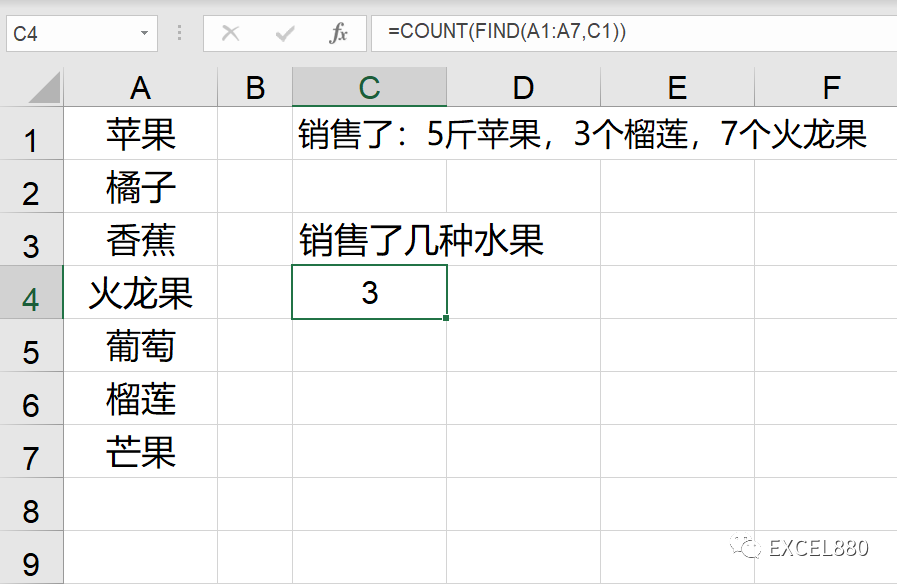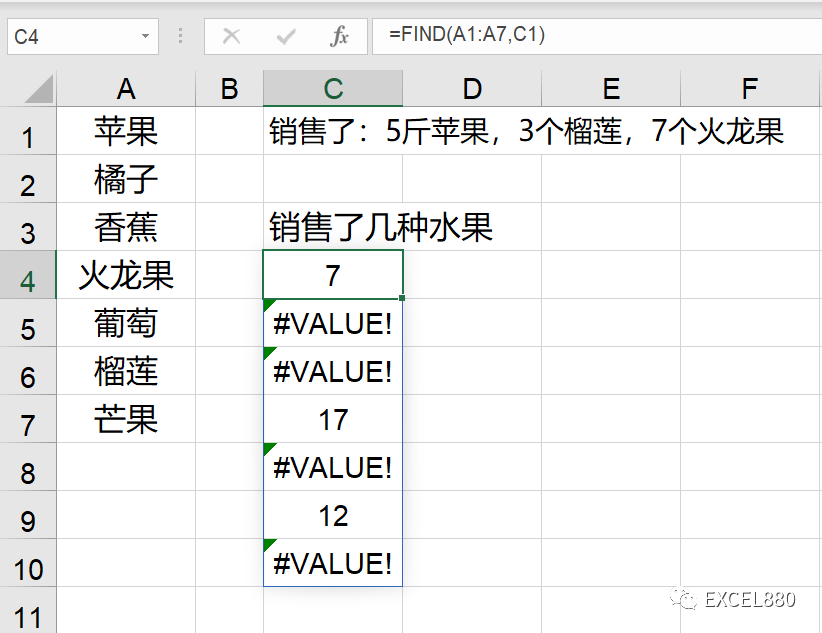# 一文详解Excel/WPS数组公式的前世今生

141 次浏览

=A1:A5Excel880笔记：数组以花括号的形式出现，也可以以连续的数据区域形式存在。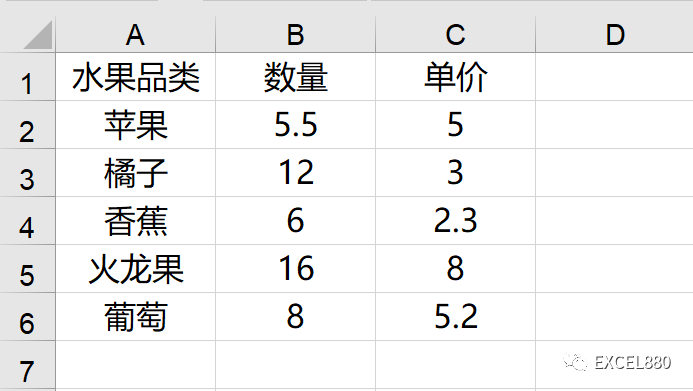=B2*C2

=SUM(D2:D6)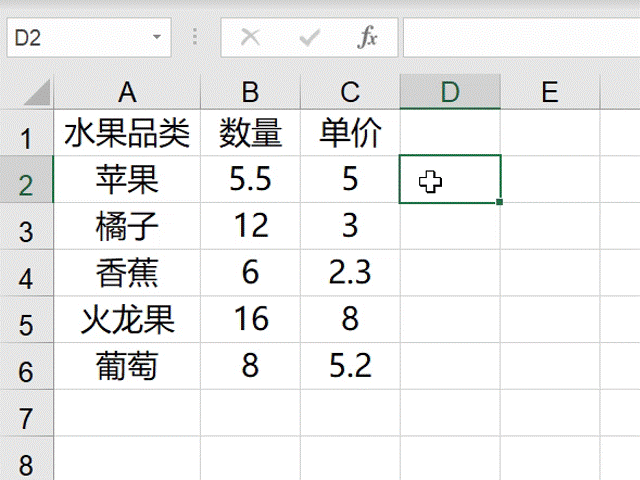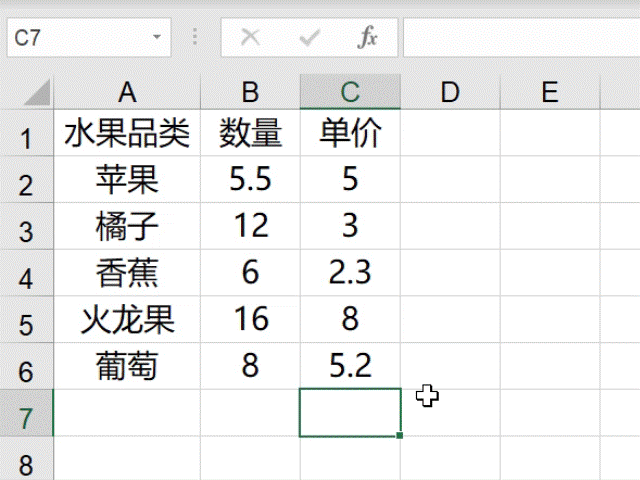=VLOOKUP(“香蕉”,A:C,{2,3},0)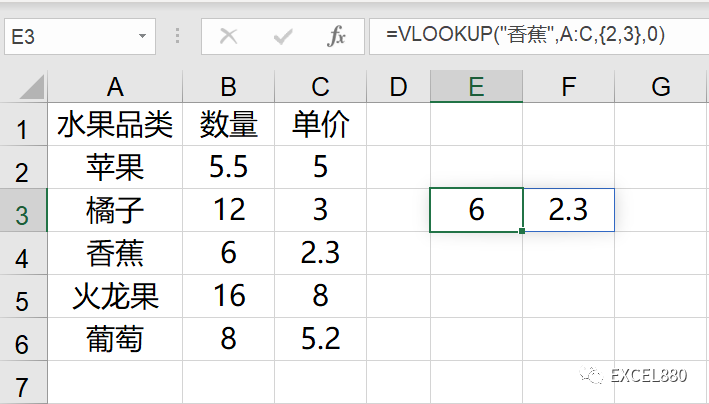=VLOOKUP({“香蕉”;”苹果”},A:C,2,0)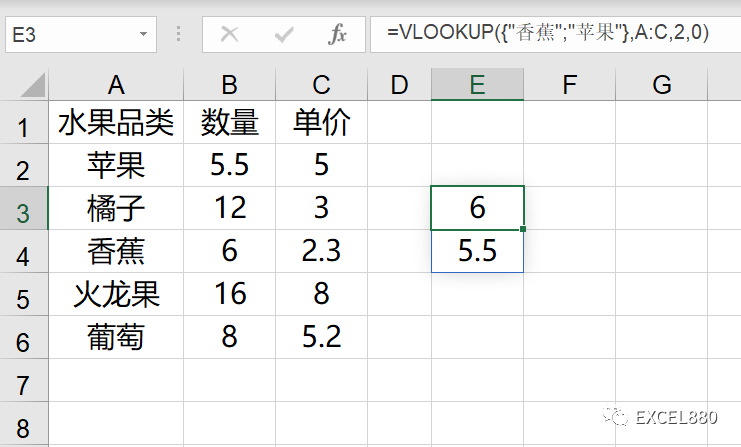{2,3}

{“香蕉”;”苹果”}

Excel笔记：数组元素之间用逗号隔开表示横向，用分号隔开表示纵向。

Excel笔记：数组元素是非数字时，要用引号包裹。

=COUNT(FIND(A1:A7,C1))